Latest Banking jobs   »

# Quantitative Aptitude Quiz For IBPS Clerk Prelims 2022- 26th July

Directions (1-10): In the given questions, two quantities are given, one as ‘Quantity I’ and another as ‘Quantity II’. You have to determine relationship between two quantities and choose the appropriate option
(a)Quantity I>Quantity II
(b)Quantity I<Quantity II
(c)Quantity I≥Quantity II
(d)Quantity I≤Quantity II
(e)Quantity I=Quantity II or no relation can’t be established.

Q1. Quantity I: Find the time taken by 120 m long train running at 72 kmph to cross the platform of length 90 m? (in sec)
Quantity II: 10 seconds

Q2. Quantity I: Rs.100
Quantity II: A shopkeeper sold an item at Rs.540 while the difference between marked price and cost price is Rs.50. Find the profit or loss, if the item was marked up by 10% above cost price? (in Rs.)

Q3. Quantity I: The probability of getting a sum, which is greater than 9, if two dices are thrown simultaneously.
Quantity II: 1/6

Q4. Quantity I: 12.5 km
Quantity II: Find the distance travelled by the boat in 2 hours in upstream, if the same boat covers a distance of 60 km in 5 hours in still water and the downstream speed is 18 kmph? (in km)

Q5. Quantity I: The ratio of ages of A to B 12 years from now will be 7:5. Find the present age of B, if A’s and B’s respective ratio of present ages is 3:1. (in years)
Quantity II: 5 years.

Q6. Quantity I: Rs. 3000
Quantity II: Find the sum if the compound interest for 2 years at the rate of 25% per annum is Rs.1687.50? (in Rs.)

Q7. Quantity I: 95
Quantity II: A man covers a total distance of ‘n’ km in 2.5 hours, out of which first half was covered at 3 kmph and second half at 6 kmph. Find the value of n²?

Q8. Quantity I: y;y²-8y-48=0
Quantity II: x;x²+12x+32=0

Q9. Quantity I: x;x²-9x+18=0
Quantity II: y;y²-13y+42=0

Q10. Quantity I: x;x²+10x-24=0
Quantity II: y;y²+5y+6=0

Direction (11-15): The following questions are accompanied by two statements i.e. statement (I) and statement (II). You have to determine which statements(s) is/are sufficient/necessary to answer the questions.
(a) Only statement I alone is sufficient to answer the question.
(b)Only statement II alone is sufficient to answer the question.
(c)Either of the statement I or statement II is sufficient to answer the question.
(d)Both the statement I and statement II together are sufficient to answer the question.
(e)Neither statement I nor statement II is sufficient to answer the question.

Q11. Find the time taken by boat to cover 80 km in still water?
Statement I: A boat takes 15 hours to cover 60 km both in upstream and downstream.
Statement II: A boat covers 30 km upstream in 5 hours while same boat covers 45 km downstream in 2.25 hours.

Q12. Find the speed of train B?
Statement I: The length of train A and train B is 120 m and 150 m respectively, whereas the respective ratio of their speed is in the ratio of 3:2.
Statement II: Train A crosses train B, which is running in opposite direction in 12 seconds.

Q13. After giving some discount to a customer, find the profit or loss acquired by the shopkeeper?
Statement I: An item was marked up by 25% and shopkeeper sold the article at Rs. 1200.
Statement II: Two successive discount of d% and 12.5% were given on the article.

Q14. Find the number of days taken by A and B to complete half of the work together?
Statement I: Number of days taken by A is 6 more than the number of days taken by B while the efficiency of A is one third of B.
Statement II: The number of days taken by A, B, C & D to complete a work is 4 days while A do same work in 24 days.

Q15. Find the total number of green balls in the bag?
Statement I: The probability of drawing one red ball from a bag containing 3 red balls, 6 blue and ‘x’ green balls is 3/11.
Statement II: The probability of drawing one green ball from a bag containing 5 white balls and remaining orange and green balls is 3/8.

Solutions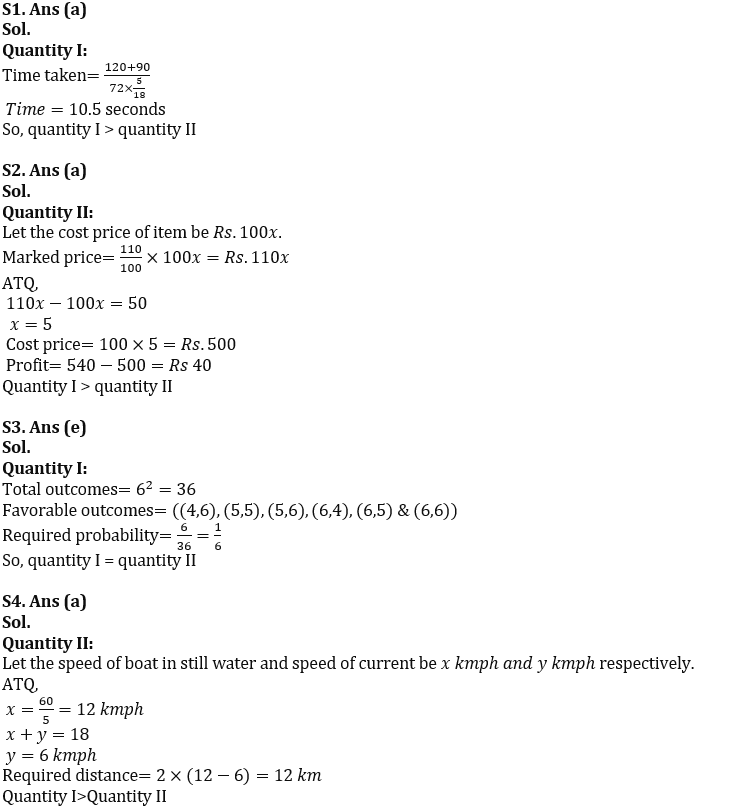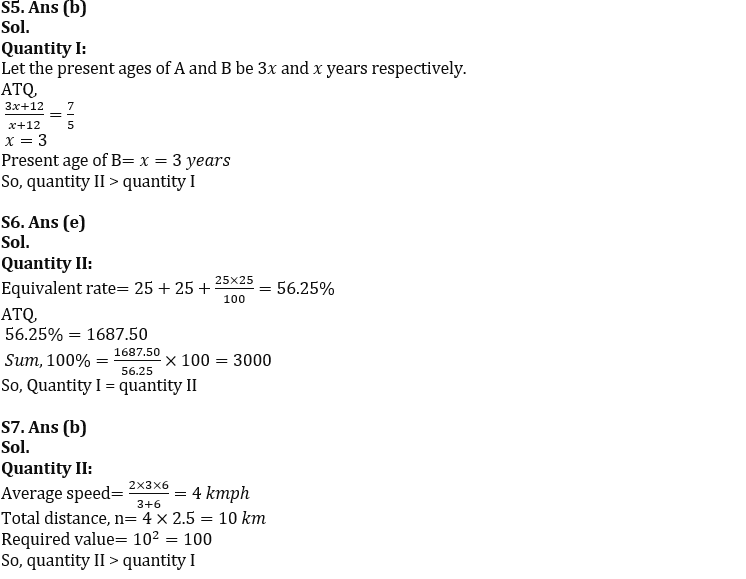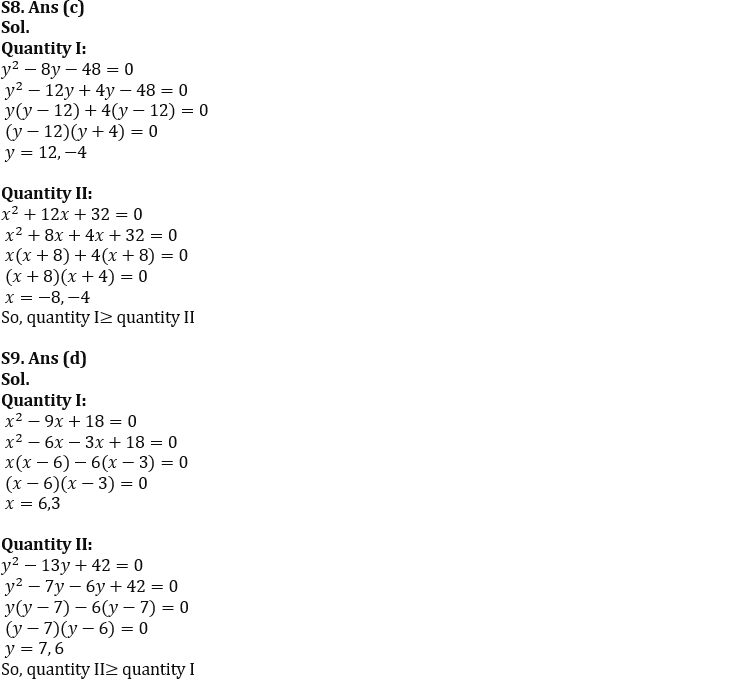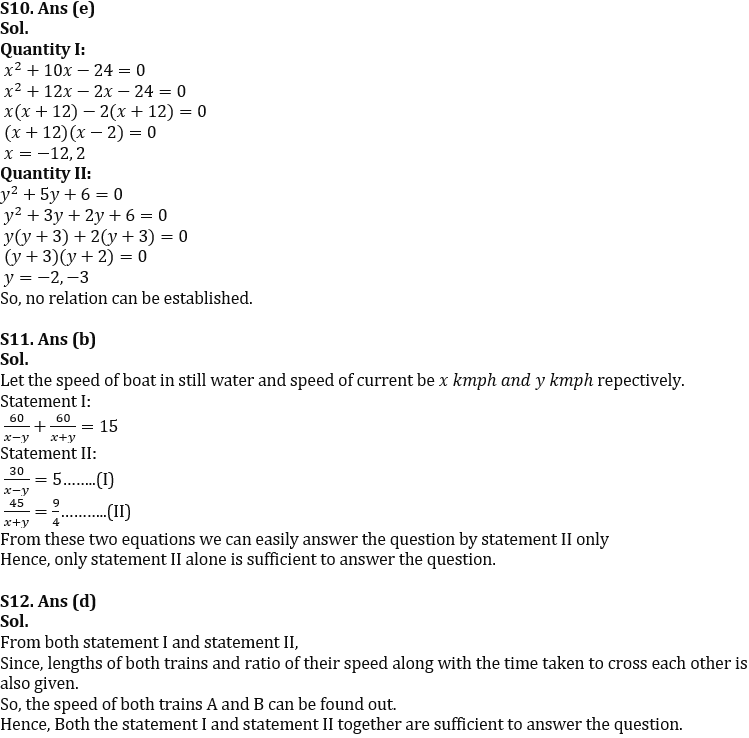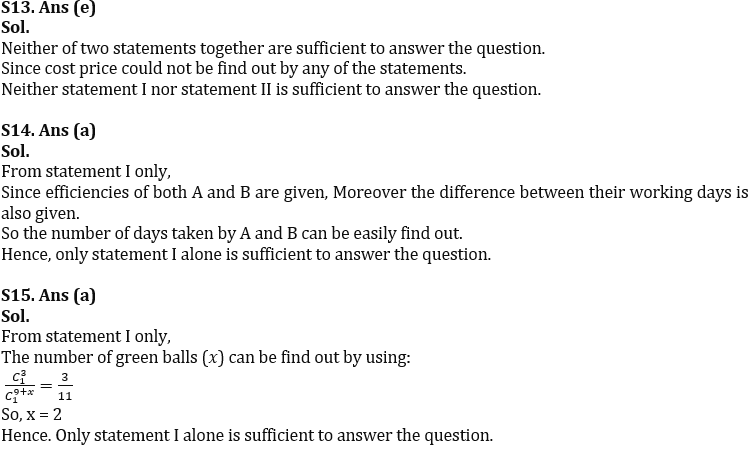#### Congratulations!